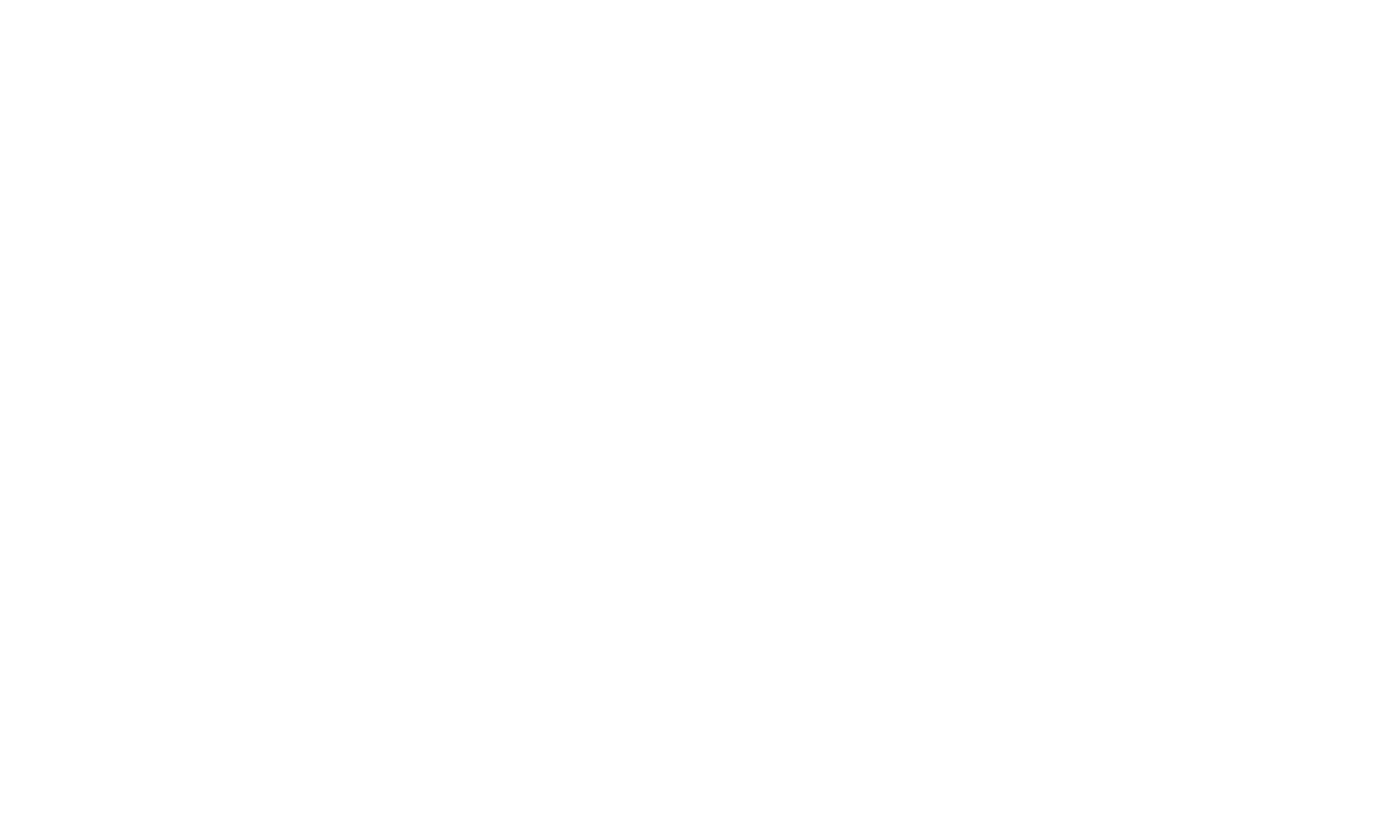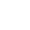## High/Low and Linear Regression Analysis

High/Low and Linear Regression Analysis Overview

The total costs associated with a business are the SUM of fixed costs and variable costs i,e. the total cost is semi-variable in nature.

• Total costs can be divided into fixed costs /variable cost per unit of output.
Formula for total costs:

Y=a+bx

where:
y= total cost in a period
a= the fixed costs in the period
b= the variable cost per unit of output or unit of activity
x= the number of output or the volume of activity in the period.

## High/Low and Linear Regression AnalysisThe total cost function can be used to estimate costs associated with different levels of activities. Its useful for forecasting and decision making.

There are two methods for constructing the total cost function equation.

1. High/Low Analysis.
2. Linear Regression Analysis.

## High/Low and Linear Regression AnalysisHigh/Low analysis;

can be used to estimate fixed costs and variable costs per unit, whenever:

• figures available for total costs at two different level of output.
• it can be used that fixed costs are same and variable cost per unit is constant at both levels of activity.
• the different between the total costs at high level and low level of output is entirely variable cost.

The following circumstances are to be considered:## When No Change

Step 1: Take activity level and cost for:

• Highest level
• Lowest level

Step 2: Calculate variable cost per unit (b) as:

difference in total cost (highest minus lowest) divide by difference in no. of units (highest minus lowest).

Step 3: Now for fixed cost (a) put the variable cost per unit into one of the cost expressions (mostly high level).

Step 4: Construct total cost function for any activity level:
Total cost=a+bx

For Example:

Step 1
Highest level 7,000 (units) costs \$38,800
Lowest level 4,500 (units) costs \$30,400

Step 2: Difference
Therefore: variable cost per unit= 8400/2500 = \$ 3.36

Step 3: Cost expressions: Total cost of 7,000 units

Fixed cost + variable cost= 38,800
Fixed cost + 7,000 x 3.36= 38,800
Fixed cost + 23,520= 38,800
Fixed cost=38,800–23,520=15,280

Step 4: Construct total cost function
Total cost=a+bx= 15,280+ 3.36x

## A step change in fixed costs as money value And the amount is known

Step 1: Take activity level and cost for:

• Highest level
• Lowest level

Step 2: Make adjustment for the step in fixed cost:

• add the step in fixed cost in lower level; or
• deduct it from higher level

Now calculate variable cost per unit (b) as:

difference in total cost (highest minus lowest) divide by difference in no. of units (highest minus lowest).

Step 3: Now for fixed cost (a) put the variable cost per unit into one of the cost expressions (mostly high level).

Step 4: Construct total cost function for any activity level:
Total cost=a+bx

For Example:

Step 1

Highest level 7,000 (units) costs \$ 38,800
Lowest level 4,500 (units) costs \$ 30,400

Step 2: Make an adjustment for step in fixed cost. For example fixed costs increase by \$3,000 when the activity level exceeds or equals 10,000 units.

Add the increase in cost in lowest activity level.

Therefore: variable cost per unit= 5400/2500 = \$ 2.16

Step 3: Cost expressions: Total cost of 7,000 units
Fixed cost + variable cost= 38,800
Fixed cost + 7,000 x 2.16= 38,800
Fixed cost + 15,120= 38,800
Fixed cost=38,800–15,120=23,680

Step 4: Construct total cost function (un-adjusted levels):

• Above 10,000 units

Total cost=a+bx= 23,680+ 2.16x

• Below 10,000 units

Total cost=a+bx=(23,680-3,000)+2.16x
Total cost=a+bx= 20,680+2.16x

## A step change in fixed costs is given as a Percentage amount

When there is a percentage change after a particular level, this means there are TWO levels which share same fixed cost.

Step 1: Take activity level and cost for (3 levels):

• Highest level
• Middle level
• Lowest level

Step 2: Choose the pair which is on the same side as the step.

Now calculate variable cost per unit (b) as:

difference in total cost divide by difference in no. of units.

Step 3: Now for fixed cost (a) put the variable cost per unit into one of the cost expressions (mostly high level).

Step 4: Construct total cost function for any activity level:

Total cost=a+bx

For Example:

Step 1

• Highest level 7,000 (units) costs \$ 38,800
• Middle level 5,500 (units) costs \$ 35,000
• Lowest level 4,500 (units) costs \$ 30,400

Step 2: Pair with same percentage change. (assume a 10% increase in fixed costs when the activity level exceeds or equals 5,500 units)

• Highest level 7,000 (units) costs \$ 38,800
• Middle level 5,500 (units) costs \$ 35,000

Therefore: variable cost per unit= 3800/1500 = \$ 2.53

Step 3: Cost expressions: Total cost of 7,000 units
Fixed cost + variable cost= 38,800
Fixed cost + 7,000 x 2.53= 38,800
Fixed cost + 17,710= 38,800
Fixed cost=38,800–17,710=21,090
Step 4: Construct total cost function (un-adjusted levels):

• Above 5,500 units

Total cost=a+bx= 21,090+ 2.53x

• Below 5,500 units

Total cost=a+bx=(21,090 x 100/110)+2.53x
Total cost=a+bx= 19,173+2.53x

## A step change in variable costs (is given as a Percentage/money amount)

• When there is a step change in variable cost per unit as money value the same approach is needed as for a step change in fixed cost. (above)
• When the change in variable cost per unit is given as a percentage amount, then variable cost(s) per unit should be calculated for TWO levels:
• variable cost per unit used above change ; and
• variable cost per unit used below change.

## High/Low and Linear Regression AnalysisIn summary, linear regression is a better technique then high/low analysis because;

• it is more reliable ; and
• it’s reliability can be measured.

Formula:

Line of best fit (y=a+bx) can be constructed by calculating values for “a” and “b” using:

a = ∑yb∑x
n       n
b= ∑xy – ∑x ∑y
n∑x2 – (∑x)2

where:

x = units
y = costs

Enter the values into the line of best fit (y=a+bx) and solve for b and then a .

The following table should be used for calculating values of “x” and “y”

Table

 X Y X2 XY

High/Low and Linear Regression Analysis